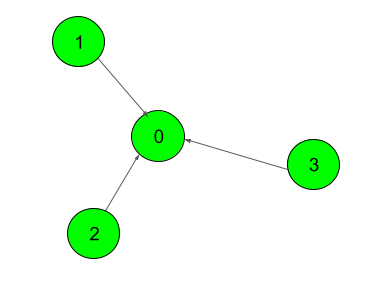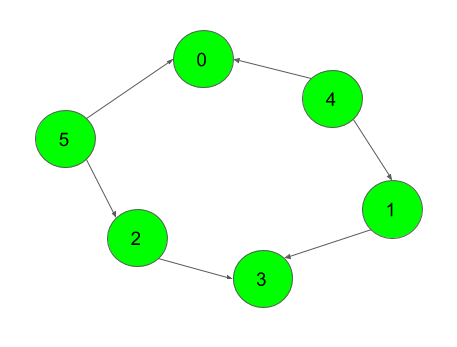XDAYS

:

HOUR

:

MINS

:

SEC

Copied to Clipboard
Topological sort
Medium Accuracy: 40.0% Submissions: 77245 Points: 4

Given a Directed Acyclic Graph (DAG) with V vertices and E edges, Find any Topological Sorting of that Graph.

Example 1:

Input:Output:
1
Explanation:
The output 1 denotes that the order is
valid. So, if you have, implemented
would be 1 for all test cases.
One possible Topological order for the
graph is 3, 2, 1, 0.

Example 2:

Input:Output:
1
Explanation:
The output 1 denotes that the order is
valid. So, if you have, implemented
would be 1 for all test cases.
One possible Topological order for the
graph is 5, 4, 2, 1, 3, 0.

You don't need to read input or print anything. Your task is to complete the function topoSort()
which takes the integer V denoting the number of vertices and adjacency list as input parameters and returns an array consisting of a the vertices in Topological order. As there are multiple Topological orders possible, you may return any of them. If your returned topo sort is correct then console output will be 1 else 0.

Expected Time Complexity: O(V + E).
Expected Auxiliary Space: O(V).

Constraints:
2
V 104
1
E (N*(N-1))/2

We are replacing the old Disqus forum with the new Discussions section given below.

Editorial

We strongly recommend solving this problem on your own before viewing its editorial. Do you still want to view the editorial?

My Submissions: